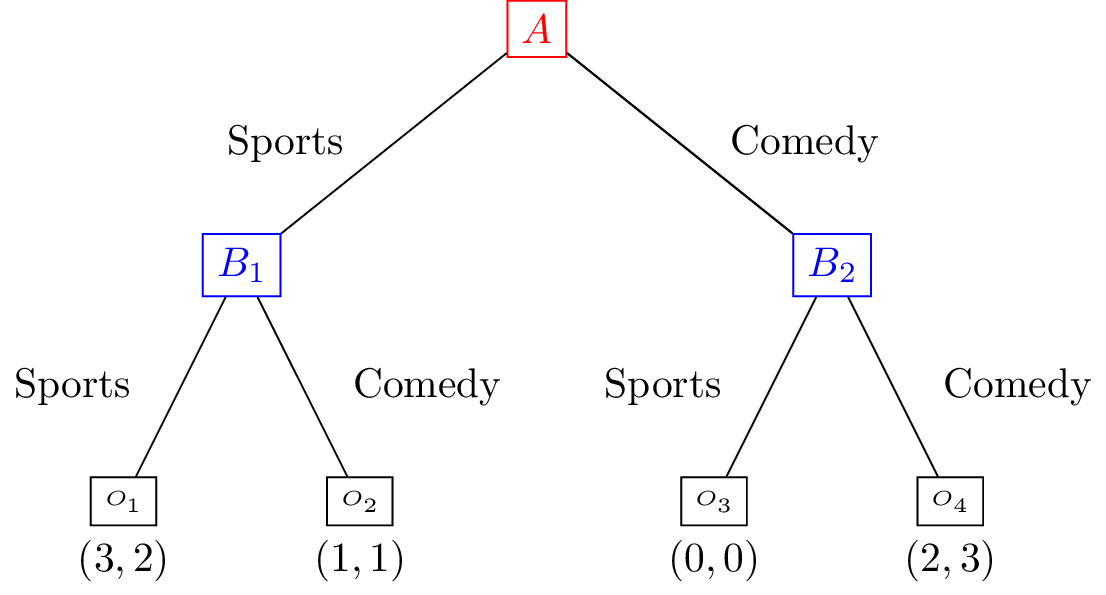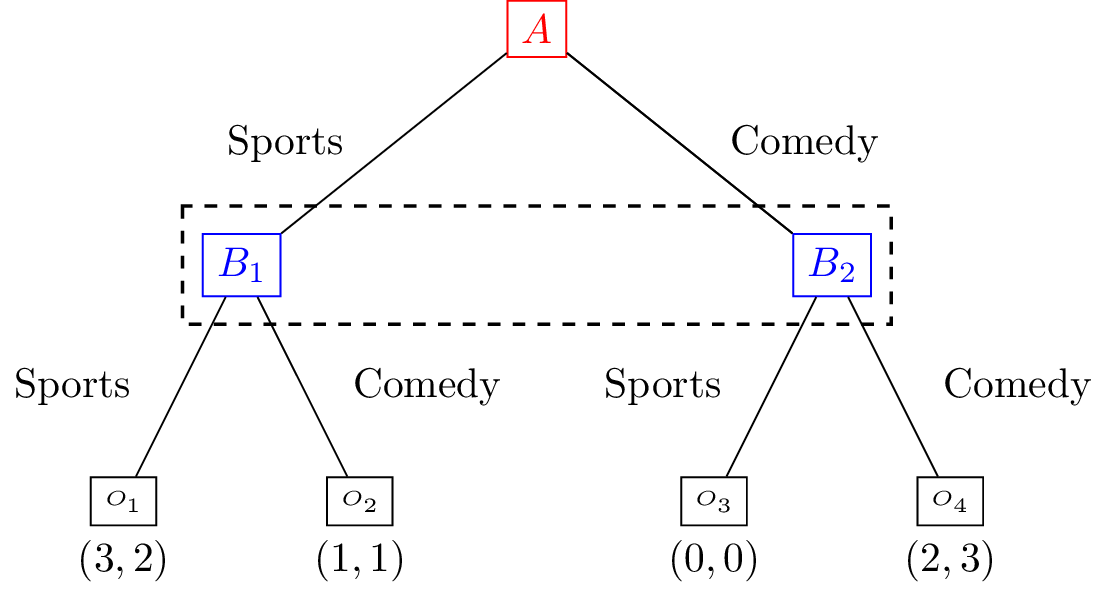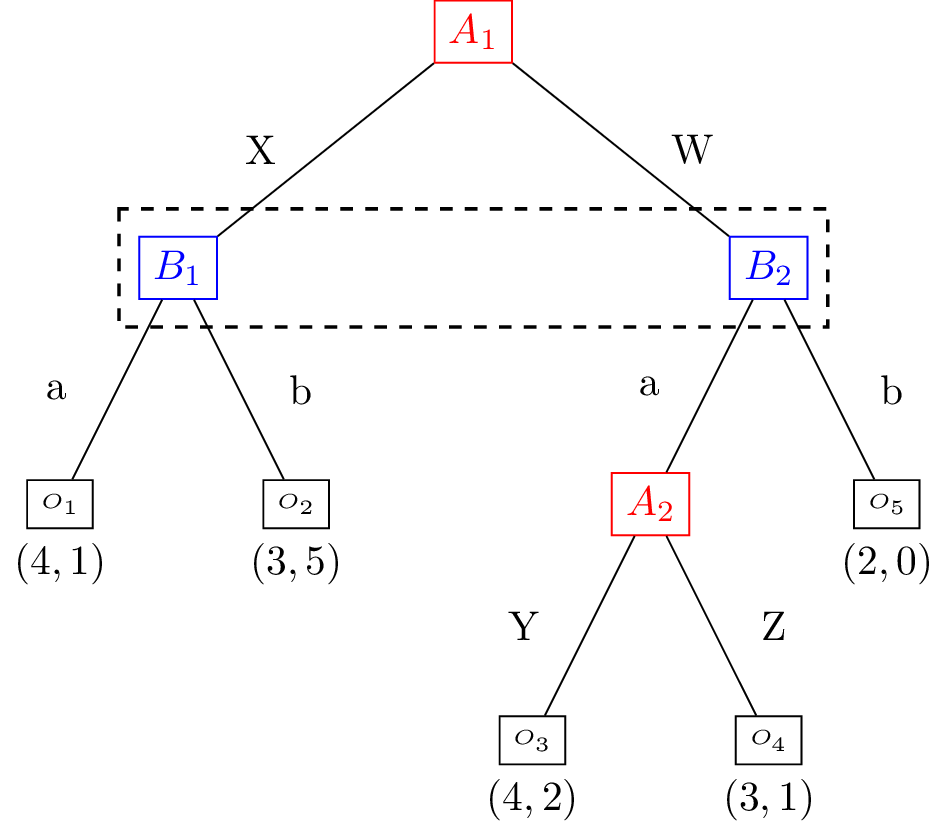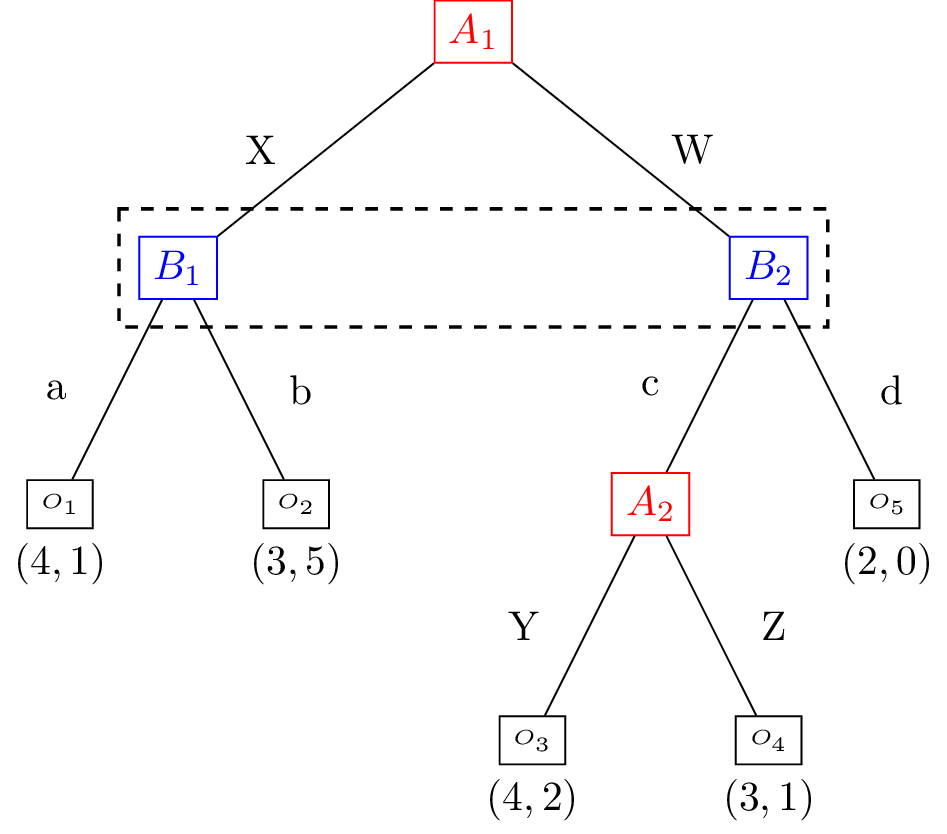# Extensive Form Games¶

## Motivating example: A modification of the Coordination Game¶

Consider the Coordination game with the modification that Alice and Bob have more information available to them: Alice decides where they are going and then lets Bob know before Bob makes their own choice.

This can be represented pictorially as follows:## Definition of an Extensive Form Game¶

An extensive form game consists of:

• A finite set of players $$\mathcal{N}$$.

• A tree: $$G = (V, E, x ^ 0)$$ where: $$V$$ is the set of vertices, $$E$$ the set of edges and $$x ^ 0 \in V$$ is the root of the tree.

• $$(V_i)_{i \in \mathcal{N}}$$ is a partition of the set of vertices that are not leaves.

• $$O$$ is the set of possible game outcomes.

• $$u$$ is a function mapping every leaf of $$G$$ to an element of $$O$$.

Question

For the modified coordination game:

1. What is the finite set of players $$\mathcal{N}$$?

2. What the elements $$G = (V, E, x ^ 0)$$?

3. What is the partition $$(V_i)_{i \in \mathcal{N}}$$?

4. What is the set of possible game outcomes $$O$$?

5. What is the mapping $$u$$ from every leaf of $$G$$ to an element of $$O$$?

## Imperfect information¶

The modified coordination game described here differs from the example given in the normal for game chapter in that Bob knows what action is chosen by Alice.

To represent imperfect information we can partition the vertices of a game tree to indicate which vertices have the same information.

This can be represented pictorially as follows:This indicates that Bob makes a decision at both nodes in $$\{B_1, B_2\}$$ without knowing at which of the two vertices they are. The set $$\{B_1, B_2\}$$ is called an information set.

## Definition of an information set¶

Given a game in extensive form: $$(\mathcal{N}, G, (V_i)_{i\in \mathcal{N}}, O, u)$$ the set of information sets $$v_i$$ of player $$i \in \mathcal{N}$$ is a partition of $$V_{i}$$. Each element of $$v_i$$ denotes a set of nodes at which a player is unable to distinguish when choosing an action.

This implies that:

• Every information set contains vertices for a single player.

• All vertices in an information set must have the same number of successors (with the same action labels).

Question

For the following games with $$\mathcal{N} = \{\text{Alice}, \text{Bob}\}$$, assume that decision nodes $$A_i$$ are Alice’s and $$B_i$$ are Bob’s. Obtain all information sets:

1.2.3.4.5.## Definition of a strategy in an extensive form game¶

A strategy for a player in an extensive form is a collection of probability distribution over the action set of each information set.

## Equivalence of Extensive and Normal Form Games¶

A game in extensive form can be mapped to a game in normal form by enumerating all possible strategies that indicate single actions at each information set. This set of possible strategies corresponds to the actions in the normal form game.

These strategies can be thought of as vectors in the space of the cross product of the sets of actions available at every information set. For player $$i\in \mathcal{N}$$ with information sets $$v_i=((v_i)_1, (v_i)_2, \dots, (v_i)_n)$$ a strategy $$s=(s_1, s_2, \dots, s_n)$$ indicates what action to take at each information set. So $$s_2$$ will prescribe which action to take at all vertices contained in $$(v_i)_2$$.

As an example consider the modified coordination game. The full enumeration of strategies that indicate single actions for Alice is:

$\mathcal{A}_1 = \{(\text{Sports}), (\text{Comedy})\}$

The full enumeration of strategies that indicate single actions for Bob is:

$\mathcal{A}_2 = \{(\text{Sports}, \text{Sports}), (\text{Sports}, \text{Comedy}), (\text{Comedy}, \text{Sports}), (\text{Comedy}, \text{Comedy})\}$

So $$(\text{Sports}, \text{Comedy})$$ indicates to choose Sports at $$B_1$$ and Comedy at $$B_2$$.

Using this enumeration the payoff functions can be given by the matrices $$A, B$$:

$\begin{split}A = \begin{pmatrix} 3 & 3 & 1 & 1\\ 0 & 2 & 0 & 2\\ \end{pmatrix}\end{split}$
$\begin{split}B = \begin{pmatrix} 2 & 2 & 1 & 1\\ 0 & 3 & 0 & 3\\ \end{pmatrix}\end{split}$

Question

Obtain the Normal Form Game representation corresponding to## Using Nashpy¶

See Solve with support enumeration for guidance of how to use Nashpy to use support enumeration to find Nash equilibria once a Normal Form game representation has been obtained.